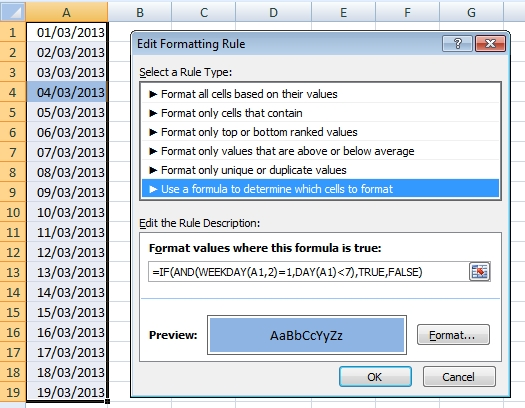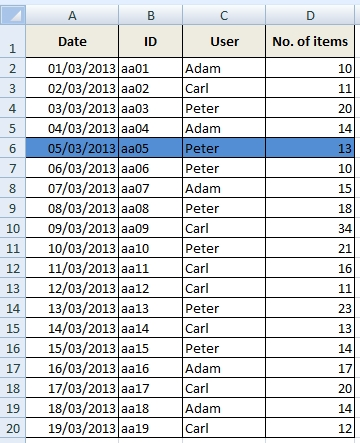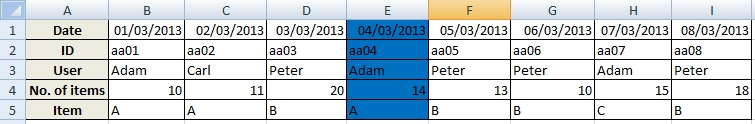# How to highlight first Monday of the month in Excel table

Let’s have a list of dates or some kind of calendar. We want to highlight first Mondays or Tuesdays or Wednesdays etc. in each month. The example shows how to use conditional formatting to solve this task.

## Highlighting the cell

Select cells with dates and go to Conditional Formatting >>  New Rule… >> Use a formula to determine which cells to format. Now write the formula and choose Format.Note: You can also select only one cell and copy conditional formatting from it to other cells (using Format Painter).

### Formula for first Monday of the month

=IF(AND(WEEKDAY(A1,2)=1,DAY(A1)<7),TRUE(),FALSE())

### Formula for Tuesday

=IF(AND(WEEKDAY(A1,2)=2,DAY(A1)<7),TRUE(),FALSE())

### Formula for Wednesday

=IF(AND(WEEKDAY(A1,2)=3,DAY(A1)<7),TRUE(),FALSE())

### Formula for Thursday

=IF(AND(WEEKDAY(A1,2)=4,DAY(A1)<7),TRUE(),FALSE())

### Formula for Friday

=IF(AND(WEEKDAY(A1,2)=5,DAY(A1)<7),TRUE(),FALSE())

### Formula for Saturday

=IF(AND(WEEKDAY(A1,2)=6,DAY(A1)<7),TRUE(),FALSE())

### Formula for Sunday

=IF(AND(WEEKDAY(A1,2)=7,DAY(A1)<7),TRUE(),FALSE())

Note that the formulas differ in only one number.

## Highlighting the whole row or column

Now we do small modifications in conditional formatting formula to highlight row and column. Place the \$ character is very important. There are the examples for Monday. Other days are similar, just change number in formula like before.

### Row / first Monday=IF(AND(WEEKDAY(\$A2,2)=1,DAY(\$A2)<7),TRUE(),FALSE())

### Column / first Monday=IF(AND(WEEKDAY(B\$1,2)=1,DAY(B\$1)<7),TRUE(),FALSE())

### Video

This video shows how to set conditional formatting for highlighting the row of table.

1.How about every other Friday? For example if in cell N3 is the date of my first paydate (1/6/2023), and my calendar is H5:N57, a conditional formatting formula to highlight the date in cell N3 in my actual calendar range and then every other friday after that.

2.I would like to do the opposite of what this formula is doing. I would like to black out all except the first Monday, Tuesday, etc. of the month. Is there a formula that will do what I need to do?

3.Do you have a formula for choosing random day? It will really solve my problem. I have a big table in Excel and I need pick any day for my calculation. Thank you.

4.Very nice function to highlight the first monday etc of the month. However, there is one problem. When the first monday is the 7th day of the month (like in Monday Sept 7 2020), it does not highlight. The Day should therefore be <8. So in my opinion the formula should read:
=IF(AND(WEEKDAY(A1,2)=1,DAY(A1)<8),TRUE(),FALSE())
Likewise for the other days of the week.

•That or <=7. Realising i'm very late to the game with this but just came across it and noticed the same error today!

5.How to choose first monday of month by conditional formatting. Nice solution. This Excel function saved my time. Thank you.

6.